# AI2 Logic

• true
• false
• not
• =
• ?
• and
• or
• ### true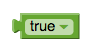Represents the constant value true. Use it for setting boolean property values of components, or as the value of a variable that represents a condition.

### false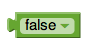Represents the constant value false. Use it for setting boolean property values of components, or as the value of a variable that represents a condition.

### not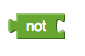Performs logical negation, returning false if the input is true, and true if the input is false.

### =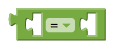Tests whether its arguments are equal.

• Two numbers are equal if they are numerically equal, for example, 1 is equal to 1.0.
• Two text blocks are equal if they have the same characters in the same order, with the same case. For example, `banana` is not equal to `Banana`.
• Numbers and text are equal if the number is numerically equal to a number that would be printed with that text. For example, 12.0 is equal to the result of joining the first character of `1A` to the last character of `Teafor2`.
• Two lists are equal if they have the same number of elements and the corresponding elements are equal.

Acts exactly the same as the = block found in Math### ?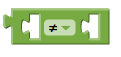Tests to see whether two arguments are not equal.

### and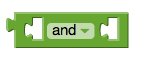Tests whether all of a set of logical conditions are true. The result is true if and only if all the tested conditions are true. When you plug a condition into the `test` socket, another socket appears so you can add another condition. The conditions are tested left to right, and the testing stops as soon as one of the conditions is false. If there are no conditions to test, then the result if true. You can consider this to be a logician's joke.

### or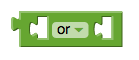Tests whether any of a set of logical conditions are true. The result is true if one or more of the tested conditions are true. When you plug a condition into the `test` socket, another socket appears so you can add another condition. The conditions are tested left to right, and the testing stops as soon as one of the conditions is true. If there are no conditions to test, then the result is false.